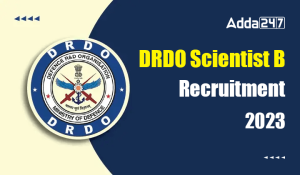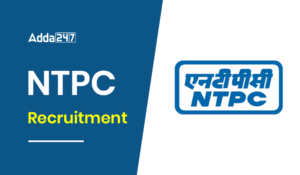Engineering Jobs   »   Mechincal Engineering quizs   »   Mechanical Engineering Quiz

# UPSSSC JE’21 ME: Daily Practices Quiz. 14-Sep-2021

Quiz: Mechanical Engineering
Exam: UPSSSC
Topic: Miscellaneous

Each question carries 1 mark
Negative marking: 1/4 mark
Time: 8 Minutes
(a) hammering
(b) casting
(c) swaging
(d) upset-forging

Q2. The temperature of wrought iron in forging is………………
(a) 12750C
(b) 5000C
(c) 9500C
(d) 1750C

Q3. The intensity of pressure at a depth h is equal to
(a) specific weight × depth
(b) specific volume × depth
(c) density × depth
(d) force depth

Q4. In PERT and CPM network the dummy activity
(a) consumes time
(b) consumes resources
(c) is used to preserve the logic
(d) is a real activity

Q5. For a single server queue, the mean arrival rate is equal to 8/hr and the mean service rate is 12/hr. The expected number in waiting line is equal to
(a) 0.5
(b) 1.33
(c) 2
(d) 3

Q6. Match List-I (Parameter) with List-II (Definition) and select answer using the codes given below the lists:
List-I
A. Time constant of a thermometer of radius r0
B. Biot number for a sphere of radius r0
C. Critical thickness of insulation for a wire of radius r0
D. Nusselt number for a sphere of radius r0
List – II
1. (hr_o)/k_fluid 2. k/h
3. (hr_o)/(3k_solid ) 4. (hπr_o l)/ρCV
Nomenclature: h: film heat transfer coefficient, ksolid: thermal conductivity of solid kfluid: thermal conductivity of fluid, ρ: density, c: specific heat, V: volume, ℓ: length
codes
A B C D
(a) 4 3 2 1
(b) 1 2 3 4
(c) 2 3 4 1
(d) 4 1 2 3

Solution

S1. Ans(d)

Sol. Large size head bolts are manufactured by the technique of UPSET FORGING.

S2. Ans(a)
Sol. Wrought iron is an alloy of iron which contain very less amount of carbon in it so it is always forged temperature below 13000C. So, option A is correct which is nearest to the 13000C.

S3. Ans.(a)
Sol. P = (ρg)h
= specific weight × height

S4. Ans.(c)
Sol. In PERT and CPM network the dummy activity is used to preserve the logic

S5. Ans.(b)
Sol.
Length of queue =(ρ^2/(1-ρ))
ρ = Utilization factor =λ/μ
Where λ = Arrival rate = 8/hr
μ = Service rate = 12/hr
∴L_q=(8/12)^2/(1-(8/12) ) = 1.33

S6. Ans.(a)
Sol.
Biot number =(hr_0)/k_solid
Nusselt number=(hr_0)/k_fluid
Critical thickness of insulation of a wire
r_0=k/h
Time constant =ρCV/hA
Where V = volume, A = area

Sharing is caring!

•GATE Result 2023 Out, Download Result, C...
•GATE 2023 Admit Card Out, Download Link ...
•IB JIO Recruitment 2023 Notification Out...
•DRDO Scientist B Recruitment 2023 Apply ...
•ISRO Scientist Recruitment 2023 Notifica...
•AP EAMCET Results 2023, Download EAPCET ...
•DIAT Recruitment 2023 Out, Apply Online ...
•NTPC Recruitment 2023, Notification, App...